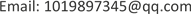——以川东南地区为例

1西南石油大学地球科学与技术学院，四川 成都

2中石化南方勘探公司勘探开发研究院，四川 成都1. 引言

2. 工区概况

3. TOC测井响应特征

4. 交会图法判别分析4.1. 交会图法原理

4.2. 交会图法优选测井参数

5. 交叉验证优选支持向量机参数

SVM判别方法的准确性直接取决于核心函数参数γ和惩罚因子C的选择。核心函数γ能够有效地将高维度的映射转变成方便的点乘，核心函数还可以定义特征空间。选择恰当的核心函数，将研究数据映射到适当的样本空间中，才能将支持向量机性能提升到高值。惩罚因子C是用来权衡损失和分类间隔的权重，因子越大从而损失越大。假如不断提高因子数值，在一定程度上会实现将样本点完全正确的分类，但又会导致过度拟合使得泛化能力不足  。

m s e = 1 N ∑ T = 1 N ( y c − y ) 2

R2是拟合优度，可以衡量回归方程整体的拟合程度，R2最大值为1，R2的值越接近1，说明回归直线对观测值的拟合程度越好，反之，R2的值越接近0，拟合程度越差。

R 2 = ∑ ( y c − y ¯ ) 2 ∑ ( y − y ¯ ) 2 或 R 2 = 1 − ∑ ( y − y c ) 2 ∑ ( y − y ¯ ) 2

6. 建立SVM回归预测模型6.1. 支持向量机与回归预测原理

6.1.1. 线性可分样本集

w * ⋅ x + b * = 0 (1)

f ( x ) = s i g n ( w * ⋅ x + b * ) 或 f ( x ) = s i g n ( ∑ i = 1 N a i * y i 〈 x i , x 〉 + b * ) (2)

min w , b = 1 2 ‖ w ‖ 2 (3)

s . t . y i ( w ⋅ x i + b ) − 1 ≥ 0 , i = 1 , ⋯ , N

6.1.2. 非线性可分样本集

min ω , b , ξ 1 2 ‖ ω ‖ 2 + C ∑ i = 1 N ξ i (4)

s . t . y i ( ω i + b ) ≥ 1 − ξ i ; i = 1 , 2 , ⋯ , N ξ i ≥ 0 ; i = 1 , 2 , ⋯ , N

f ( x ) = s i g n ( ∑ i − 1 N a i * y i k ( x i , x ) + b * ) (5)

6.2. 支持向量机模型构建

f ( x ) = ω ⋅ ϕ ( x ) + b = ∑ i = 1 n ( a i * − a i ) K ( x i ⋅ x ) + b * (6)

ω ——权数；

ϕ ( x ) ——非线性映射函数集合；

b——阈值；

a i * 、 a i 、b——可通过某点数值计算得到的模型参数 K ( x i ⋅ x ) 为核函数且满足下式：

K ( x i ⋅ x ) = exp ( 1 − | x − x i | 2 σ 2 ) (7)

6.3. 训练集和测试集回归预测结果精度图

7. 应用实例分析

8. 结论

1) 训练集与测试集的测试结果相差不大，且拟合度普遍较高，平均平方误差小，但对于含量偏低的数据测试不佳，测试模型还有所欠缺。

2) 经过实验测试，利用支持向量机拟合一定范围内的TOC值有较高的准确率，支持向量机不失为探测TOC值的新方法。